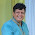## Wednesday, August 1, 2018

Geometry Problem. Post your solution in the comment box below.
Level: Mathematics Education, High School, Honors Geometry, College.

Details: Click on the figure below.1.Let:
r_1 =sqrt(3)a
r_2=sqrt(3)b
h=sqrt(3)c

Let R be the point on the line AB where the circle of radius r_1 is tangent.
Let T be the point on the line AB where the circle of radius r_2 is tangent.
Let N be the point where the circle of radius r_2 is tangent to the extension of DC
Let O_1 be the center of the circle radius r_1.

If we let M be the point on DN where the circle O_1 is tangent then O_1RAM is cyclical.
Because ∠MAR =120 the triangle ARO_1 is a 30-60-90 triangle and AR=a

If h=sqrt(3)c then the length of the sides of the equilateral triangle ABC =2c ,therefore AB =2c and BR =2c-a

Be similar reasoning we find that AT=b and BT =2c-b

Then let Q be the point where the circle of radius r_2 is tangent to the extension of line DB and let P be the point where the circle of radius r_1 is tangent to the line DB.

Then PB = BR and BQ=BT therefore

PB =2c -a
BQ=2c-b

and

PQ=PB+BQ
PQ= 4c-a-b

We also have MA =a ,AC =2c and CN =b therefore

MN=MA+AC+CN
MN=2c+a+b

MN=PQ (common tangent line) therefore

4c-a-b=2c+a+b
2c=2a+2b
c=a+b

With r_1=sqrt(3)a,r_2=sqrt(3)b and h=sqrt(3)c we have

h/sqrt(3)=a/sqrt(3)+b/sqrt(3)
h=r_1+r_2

1.I noticed i made an error in the last two lines.

h/sqrt(3)=a/sqrt(3)+b/sqrt(3)

it should have been :

h/sqrt(3)=r_1/sqrt(3)+r_2/sqrt(3)

Then we get

h=r_1+r_2

2.Let in circle be P and excircle be Q.
Let DAC touch circle P at X and circle Q at Y
Let DB touch circle P at U and circle Q at V

Let AB = BC = CA = a
Let AX = b and CY = c

UV = XY so a-b + a-c = b + a + c
So b + c = a/2 ......(1)

But b = r1/sqrt3 and c = r2/sqrt3
Further a/2 = h/sqrt3

So from (1),

r1 + r2 = h

Sumith Peiris
Moratuwa
Sri Lanka

1.Such an elegant solution. After having tried for pretty long time I gave up and checked the solution. You make it look so simple and easy.
Kudos to you Sir ......!!

3.Let the center of the circle with radius r1 be P and meet DA at E and similary the center of r2 be Q and meet at F
Let the length of equilateral triangle be 'a'
Let the length of DE be 'x'
QCF is 30-60-90 triangle, hence CF=r2/sqrt(3) ------------(1)
Also PAE is 30-60-90 triangle, hence EA=r1/sqrt(3) ------------(2) (Since m(c)=60,m(B)=60=>m(DAB)=120)
As we know DF=Semi-perimeter of triangle DBC=DE+EA+AC+CF
=> S = x+r1/sqrt(3)+a+r2/sqrt(3)-------------------(3)

However S=(DB+BC+CD)/2
=> S=[(x+a-r1/sqrt(3))+(a)+(a+r1/sqrt(3)+x)]/2
=> S=x+3a/2 ----------------(4)

As (3)=(4)
=> r1/sqrt(3)+r2/sqrt(3)=a/2
=> r1+r2=h Q.E.D

4.See the drawing

Define a=AB=BC=AC

DC and DB tangents to Circle O1 => DG=DE=b
AD and AB tangents to Circle O1 => AG=AJ=d
DB and BA tangents to Circle O1 => BE=BJ=c

DB and BC tangents to Circle O2 => BF=BK=e
DC and BC tangents to Circle O2 => CI=CK=f

a=d+c=e+f
DF=b+c+e, DI=b+d+a+f
DC and DB tangents to Circle O2 => DF=DI
=> b+c+e=b+d+a+f => (a-d)+(a-f)=d+a+f
Therefore a=2d+2f

∠BAC=Π/3, ∠O1AG=∠O1AJ => ∠O1AG=∠O1AJ =Π/3
=>ΔAGO1 is similar to ΔAHB
In the same way ΔCIO2 is similar to ΔAHB

h=NB, a=AB=BC=AC => a=2h/sqrt(3)
In the same way, AG=d=r1/sqrt(3) and CI=f=r2/sqrt(3)

a=2d+2f => 2h/sqrt(3) =2 r1/sqrt(3) + 2 r2/sqrt(3)
Therefore h=r1+r2

5.The use of sqrt(3) can be avoided.
The similarity of the 3 triangles and the fact that their sides on AC add-up give the required result.